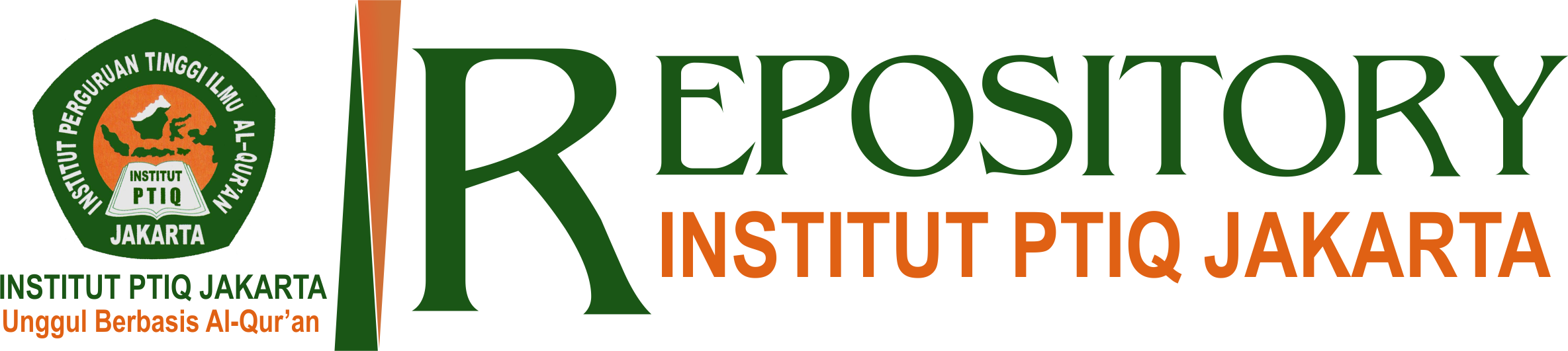#### Kreativitas Guru, Manajemen Kelas Dan Prestasi Belajar Siswa Pada Mata Pelajaran PAI (Studi Kasus di SMPIT Global Insani Islamic School Bekasi)

Sarnoto, Ahmad Zain (2018) Kreativitas Guru, Manajemen Kelas Dan Prestasi Belajar Siswa Pada Mata Pelajaran PAI (Studi Kasus di SMPIT Global Insani Islamic School Bekasi). Madani Institute: Jurnal Politik, Hukum, Pendidikan, Sosial, dan Buadaya, 7 (2). ISSN 2776-978X

## Abstract

This event will be based on research by the important role carried out by PAI teachers to improve learning achievements of students in the subjects of PAI. This research includes research of korelasional consisting of three variables, namely creativity guru (X 1) and management classes (X 2) as a free variable, and learning achievements of students (Y) as variables associated with it. This research aims to find out whether there is influence the creativity of teachers and classroom management learning achievements against the students, either separately or jointly.

The population of this research is the entire Global Human SMPIT student Islamic School in Bekasi, with a total population of 178 students. As for how the withdrawal of samples in this research by using the techniques of Probability Sampling. Instrument in the collection of data on two free variables using questionnaire (question form). Data analysis techniques and results of research using the SPSS Statistical Software regression and correlation.

The results of this research in general i.e. There are positive and significant influence teachers ' creativity and management classes against the achievements of the student learning. As for those special conclusions in detail are as follows: based on the analysis of the data it can be concluded that the author do first: (1) there is a positive and significant influence teachers ' creativity toward accomplishments of the correlation coefficient with learning (ryx1) is 0.633 and the coefficient of determination R2 (R square) = 0.400, meaning that the creativity of teachers towards learning achievements amounted to 40.0% and the rest IE 60% is determined by other factors. As for the direction of regression equations (unstandardized coefficients B) Ŷ = 15.524 0.544 + X 1, which means that every one unit increase in score creativity guru will influence the improvement of learning achievement score 0,544., (2) there is a significant and positive influence on the management of class learning achievements against with the coefficient of correlation (ryx1) is 0.611 and the coefficient of determination R2 (R square) = 0.374, which means that the management of class learning achievements against amounted 37.4% and the rest IE 62.6% determined by other factors. As for the direction of regression equations (unstandardized coefficients B) Ŷ = 18.618 + 0.529 x 2, which means that each increase of one unit of score management class will affect the improvement of the learning achievement score of 0.830., (3) there is a positive and significant influence teachers ' creativity and management of class learning achievements against together with correlation coefficients (ryx1) is 0.611 and (ryx2) is 0.814 with the coefficient of determination R2 (R square) = 0.428 , which means that the creativity of teachers and classroom learning achievements against management of 42.8% and 57.2% i.e. the rest is determined by other factors. As for the direction of regression equations (unstandardized coefficients B), Ŷ = 10.825 0.344 + x 1 + X 2, 0.247 which means that every one unit increase in score creativity teacher and class management will affect the improvement of the learning achievement score of 0.591

Item Type: Article 300. Ilmu Sosial > 370. Pendidikan Jurnal Online Institut PTIQ Jakarta Andi Jumardi 04 Dec 2022 04:49 04 Dec 2022 04:49 https://repository.ptiq.ac.id/id/eprint/846